• 主要介绍了python简单实现最大似然估计&scipy库的使用详解，具有很好的参考价值，希望对大家有所帮助。一起跟随小编过来看看吧
• 1. 什么是最大似然估计？...最大似然估计是使用概率模型、利用已知的样本结果，反推一件事情最有可能发生（最大概率）导致这样结果的参数值，是一类完全基于统计的系统发生树重建方法的代表，可以理解为样本所展现的...
1.	什么是最大似然估计？

在一次机器语言的学习中，引入了一个新概念—最大似然估计。然而面对繁琐的数学公式和复杂的推理过程，使我对概念非常模糊，也不懂到底是什么原理，但通过后来的慢慢学习使我对最大似然估计有了不一样的认识。 最大似然估计是使用概率模型、利用已知的样本结果，反推一件事情最有可能发生（最大概率）导致这样结果的参数值，是一类完全基于统计的系统发生树重建方法的代表，可以理解为样本所展现的是所有可能状态中概率最大的状态。这种对复杂问题概念的求解问题使我联想到贝叶斯公式，在很多求解概率的问题上都离不开它，由此，从贝叶斯公式与最大似然估计两者之间的联系和区别又对最大似然估计方法又有了更深刻的认识。最大似然估计方法为什么受欢迎？他和贝叶斯公式之间最大的不同在哪？最大似然方法估计的原理是什么？既然是通过概率模型进行求解那么在实际问题中应该选用那种模型呢？最大似然估计方法的应用有哪些？ 2. 问题分析 在概率论中，了解到贝叶斯公式是一个后验概率，什么是后验概率？简单来说，后验概率是基于“结果”的信息重新修正后的概率，所获得的概率更接近实际情况。但是在实际应用中，样本数据不多，特征值的维度也不够大导致直接计算条件概率的难度较大，所以最大似然估计方法相当于贝叶斯公式的升级版，将概念密度估计转化成了参数估计，在样本数量无限多时，结果会更接近于真实值，这对我们日常生活中处理概率问题有很大帮助，这也是为什么最大似然估计这么受欢迎的原因。 同时，两者最大的区别也就出现了，那就是参数。非常能够总结最大似然估计的一句话是“模型已定，参数未知”，通过若干次试验观察其结果，得到某个参数值使样本出现的概率最大，是一种在实际问题求解中的简化方法。最大似然估计方法是建立在最大似然原理之上的，极大似然估计是建立在极大似然原理的基础上的一个统计方法，如果单从概念不能完全理解，就用一个简单的例子来介绍最大似然原理：
有两个形状大小一样的箱子里面各有10个球，第一个箱子里面有2个红球8个篮球，另一个箱子里有8个红球2个篮球，当问你觉得红色球是从哪个箱子里拿出来的时候，人们都会觉得是从第二个箱子里面拿出来的，这个推断符合日常生活中的经验事实，而最大可能就是最大似然之意，这种想法也被称为最大似然原理。这样理解起来是不是就会更容易！
接下来，就是最大似然估计模型的推导过程： 假设样本集D={x1 、x2 、…、xn}，假设样本之间都是相对独立的，注意这个假设很重要！于是便有：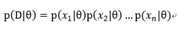所以假设似然函数为：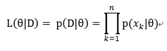接下来我们求参的准则便是如名字一样最大化似然函数喽：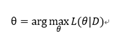引入拉普拉斯修正，也就是取对数ln，所以做出下面变换：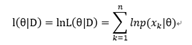所以最大化的目标便是：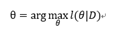再使用数学中的微分知识求解：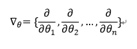最后使偏导数为0：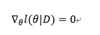由此得到参数θ，求出类条件概率密度进行判别 通过对似然估计的推导，是不是对其有更清晰的认识了呢~
应用 大家肯定会问，最大似然估计到底有什么用？ 其实最大似然估计在科学、医疗、经济等领域上都有广泛的应用 例如： 求取广义线性模型之最大似然估计： 应用于求取乳腺癌死亡率之小区域估计 最大似然估计在保险精算中的应用研究 最大似然估计在GPS定位中的应用研究 最大似然估计的信息融合方法及其在水下航行器制导中的研究 关于截尾样本情形下的最大似然估计 基于最大似然估计的雷达高度计跟踪处理 广义最大似然估计在OCT无创血糖检测中的应用  总结 求最大似然估计量的一般步骤： （1）写出似然函数； （2）对似然函数取对数，并整理； （3）求导数； （4）解似然方程。
这篇文章对最大似然估计的概念以及基本推导过程进行叙述和展示，希望对一样有需求的人有帮助，最大似然估计的应用广泛，比其他估计方法更简单，当样本数量较多时收敛性也会非常好，在应用中需要结合特定的环境，因为它需要你提供样本的已知模型进而来估算参数，假设类条件概率模型正确，通常都能获得较好的结果。
参考文献：《应用概率统计》
展开全文•matlab
• 极大似然估计方法估计方法，极大似然估计方法估计方法
• 我们的方法称为最大似然互信息（MLMI），具有几个有吸引力的特性，例如不涉及密度估计，它是一个单一的过程，全局最优解可以有效地计算，并且交叉验证可用于模型选择。数值实验表明，MLMI与现有方法相比具有较好的...
• 概率论与数理统计——最大似然估计的计算题目最大似然估计使用最大似然估计的解题步骤第一种情况：离散型① 选择样本/样本值（准备工作）② 构造似然函数（重点）③ 求导（收尾工作）总结第二种情况：连续型① 选择...


概率论与数理统计——最大似然估计的计算
题目最大似然估计使用最大似然估计的解题步骤第一种情况：离散型① 选择样本/样本值（准备工作）② 构造似然函数（重点）③ 求导（收尾工作）总结
第二种情况：连续型① 选择样本/样本值（准备工作）② 构造似然函数（重点）③ 求导（收尾工作）总结
两种情况对比
答案

题目
eg：设

X

1

,

X

2

.

.

.

X

n

X_{1},X_{2}...X_{n}

是来自总体

X

X

的一个样本，

X

∼

B

(

m

,

p

)

X \sim B(m,p)

，其中

m

m

已知，试求参数

p

p

的最大似然估计(答案在文末)。
最大似然估计
对于点估计，有矩估计法和最大似然估计法。
矩估计法，其基于大数定律，求解未知参数

θ

θ

的时候，是一种简单的替换的思想（样本矩估计总体矩）。   最大似然估计法，基于极大似然原理（概率大的事件在一次观测中更容易发生）。求解未知参数

θ

θ

的时候，是当它作为估计值时，使样本出现的概率（样本出现的可能性）最大。

矩估计可以使用在总体分布未知的情况，但最大似然估计必须要知道总体的分布。因此，矩估计具有简单的优点，但是当已知分布的时候，使用矩估计就会放弃这一已知信息，从而对于我们估计出的值造成误差，为了充分利用已知的信息，我们需要使用最大似然估计。

使用最大似然估计的解题步骤
第一种情况：离散型
① 选择样本/样本值（准备工作）
从总体中选取样本或者样本值，在这里我们选择样本值

x

1

,

x

2

.

.

.

x

n

x_{1},x_{2}...x_{n}

。   因为我们知道总体

X

X

的分布，因此，对于从

x

1

x_{1}

到

x

n

x_{n}

中的任意一个值，都可以代入

X

X

的分布中，求出当

X

X

等于该值的概率，将这些概率分别记成

P

1

,

P

2

.

.

.

P

n

P_{1},P_{2}...P_{n}

，这些概率，都是包含了

i

(

x

i

,

i

=

1

,

2...

n

)

i(x_{i},i=1,2...n)

和未知参数

θ

θ

的函数。这就为我们构造似然函数做了准备（在做题时可省略这一步，心中有数即可）。
② 构造似然函数（重点）
准备工作结束，我们在准备中已经得到了

P

1

,

P

2

.

.

.

P

n

P_{1},P_{2}...P_{n}

，将这些概率相乘，就得到了我们的

L

(

θ

)

L(θ)

。这些

P

P

，其中都包含了未知的

θ

θ

参数，对于不同的题目，

θ

θ

可以是

μ

μ

，

p

p

等。这个

L

(

θ

)

L(θ)

，又叫样本的联合分布律。   简而言之，构造

L

(

θ

)

L(θ)

，就是将每一个样本值对应的概率相乘。   上面就构造出了

L

(

θ

)

L(θ)

，但对于大部分题目，这个

L

(

θ

)

L(θ)

往往很复杂，因此，我们需要将其取对数（取

l

n

ln

值），便于我们之后的求导计算。
③ 求导（收尾工作）
我们已经构造出了似然函数且取了对数简化计算，接下来，就是对这个简化了的函数进行求导，求导的目的是为了得到导数等于零的点，这一点往往就是似然函数取最大值的点。之前我们说过，最大似然估计就是在

θ

θ

作为估计值时，样本出现可能性取最大，也就是似然函数取最大，因为似然函数就是每一个点的出现概率的乘积。因此，我们通过求导，令导数等于零，就能够求出令似然函数最大的

θ

θ

值了。
总结
因此，离散型总体最大似然估计法的步骤为：选择样本值→构造似然函数(每个样本值对应概率相乘)→似然函数取对数(方便计算)→求导→令导数为0→求出未知参数θ的最大似然估计值
第二种情况：连续型
因为和离散型的类似，因此简略叙述。
① 选择样本/样本值（准备工作）
依旧是选取样本或者样本值，在这里我们选择样本值

x

1

,

x

2

.

.

.

x

n

x_{1},x_{2}...x_{n}

。   因为我们知道总体

X

X

的分布，因此我们知道总体

X

X

的概率密度函数

f

(

x

)

f(x)

，因此，我们将

x

1

x_{1}

到

x

n

x_{n}

分别带入概率密度函数中，得到

f

1

(

x

)

,

f

2

(

x

)

.

.

.

f

n

(

x

)

f_{1}(x),f_{2}(x)...f_{n}(x)

，它们都包含了未知参数

θ

θ

。准备工作完成。
② 构造似然函数（重点）
准备工作结束，我们在准备中已经得到了

f

1

(

x

)

,

f

2

(

x

)

.

.

.

f

n

(

x

)

f_{1}(x),f_{2}(x)...f_{n}(x)

，将它们相乘，就得到了我们的

L

(

θ

)

L(θ)

。这个

L

(

θ

)

L(θ)

，又叫样本的联合概率密度。   取对数，便于求导。
③ 求导（收尾工作）
求导，令导数等于零，求出令似然函数最大的

θ

θ

值。
总结
因此，连续型总体最大似然估计法的步骤为：选择样本值→构造似然函数(每个样本值对应概率密度函数相乘)→似然函数取对数(方便计算)→求导→令导数为0→求出未知参数θ的最大似然估计值
两种情况对比
离散型和连续型唯一的区别，就是离散型取的是每一个样本点的概率，而连续型取的是每一个样本点的概率密度。它们都包含了参数

θ

θ

，都可以通过取对数求导来算出最大似然估计值。
答案

似然函数为

L

(

p

)

=

∏

i

=

1

n

P

(

X

i

=

x

i

)

=

∏

i

=

1

n

C

m

x

i

p

x

i

(

1

−

p

)

m

−

x

i

=

p

∑

i

=

1

n

x

i

(

1

−

p

)

∑

i

=

1

n

(

m

−

x

i

)

∏

i

=

1

n

C

m

x

i

L(p)=\prod_{i=1}^n P(X_{i}=x_{i})=\prod_{i=1}^n C_{m}^{x_{i}}p^{x_{i}}(1-p)^{m-x_{i}}=p^{\sum_{i=1}^n x_{i} }(1-p)^{\sum_{i=1}^n (m-x_{i}) }\prod_{i=1}^n C_{m}^{x_{i}}

取对数得

l

n

L

(

p

)

=

∑

i

=

1

n

x

i

l

n

p

+

∑

i

=

1

n

(

m

−

x

i

)

l

n

(

1

−

p

)

+

l

n

∏

i

=

1

n

C

m

x

i

lnL(p)=\sum_{i=1}^n x_{i} lnp+\sum_{i=1}^n (m-x_{i})ln(1-p)+ln\prod_{i=1}^n C_{m}^{x_{i}}

求导

d

l

n

L

(

p

)

d

p

=

∑

i

=

1

n

x

i

p

−

∑

i

=

1

n

(

m

−

x

i

)

1

−

p

\frac{dlnL(p)}{dp}=\frac{\sum_{i=1}^n x_{i} }{p}-\frac{\sum_{i=1}^n (m-x_{i})}{1-p}

令

d

l

n

L

(

p

)

d

p

=

0

\frac{dlnL(p)}{dp}=0

解得

p

^

=

1

m

n

∑

i

=

1

n

x

i

=

1

m

x

ˉ

\hat{p}=\frac{1}{mn}\sum_{i=1}^n x_{i} =\frac{1}{m}\bar{x}


展开全文• Matlab2016a实验内容：给定以下w1和w2的三维数据，考虑不同维数下的高斯概率密度模型：（a）编写程序，对表格中的类w1中的3个特征，分别求解最大似然估计。 （b）修改程序，处理二维数据的情形。然后处理对表格中的...
机器学习实验三，详情请参考《模式分类》第二版第三章课后上机练习3.2节
实验环境：
Matlab2016a
实验内容：
给定以下w1和w2的三维数据，考虑不同维数下的高斯概率密度模型：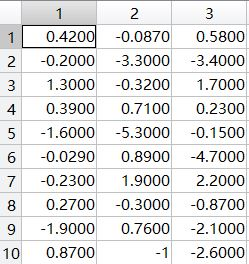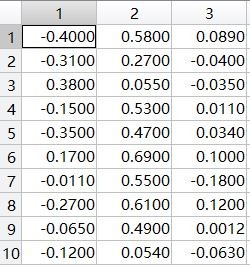（a）编写程序，对表格中的类w1中的3个特征，分别求解最大似然估计。  （b）修改程序，处理二维数据的情形。然后处理对表格中的类w1中的任意两个特征的组合（3种可能）。  （c）修改程序，处理三维数据的情形。然后处理表格中类w1中3个特征的组合。  （d）假设这个三维高斯模型是可分离的，即，写一个程序估计类别w2中均值和协方差矩阵的三个参数。
实验步骤：
在课本（模式分类第二版第三章）中，通过将似然函数最大化，我们可以得到以下针对高斯分布下的最大似然估计：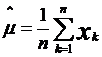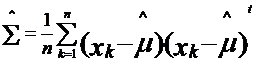我们可以直接利用这俩公式来求每个特征的最大似然估计。对于（a），分别三种一维情况：
load sample_ex3.mat;
x1=w1(:,1);x2=w1(:,2);x3=w1(:,3);
u1=mean(x1);sigma1=mean((x1-u1).^2);
u2=mean(x2);sigma2=mean((x2-u2).^2);
u3=mean(x3);sigma3=mean((x3-u3).^2);
fprintf('the mean and variance of feasure x1: %f ,%f \n',u1,sigma1);
fprintf('the mean and variance of feasure x2: %f ,%f \n',u2,sigma2);
fprintf('the mean and variance of feasure x3: %f ,%f \n',u3,sigma3);
运行结果如下：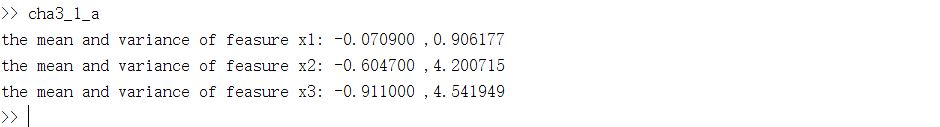对于（b），同样利用上述公式，只是情况变为二维：
load sample_ex3.mat;
x12=[w1(:,1) w1(:,2)];x13=[w1(:,1) w1(:,3)];x23=[w1(:,2) w1(:,3)];
u12=mean(x12',2);u13=mean(x13',2);u23=mean(x23',2);
sum12=0;sum13=0;sum23=0;
for i=1:size(u12,1)
sum12=sum12+(x12(i,:)'-u12)*(x12(i,:)'-u12)';
end
for i=1:size(u13,1)
sum13=sum13+(x13(i,:)'-u13)*(x13(i,:)'-u13)';
end
for i=1:size(u23,1)
sum23=sum23+(x23(i,:)'-u23)*(x23(i,:)'-u23)';
end
sigma12=sum12/size(u12,1);
sigma13=sum13/size(u13,1);
sigma23=sum23/size(u23,1);
disp('the mean and covariance of feasure x1 and x2:');
disp(u12);disp(sigma12);
disp('the mean and covariance of feasure x1 and x3:');
disp(u13);disp(sigma13);
disp('the mean and covariance of feasure x2 and x3:');
disp(u23);disp(sigma23);
运行结果如下：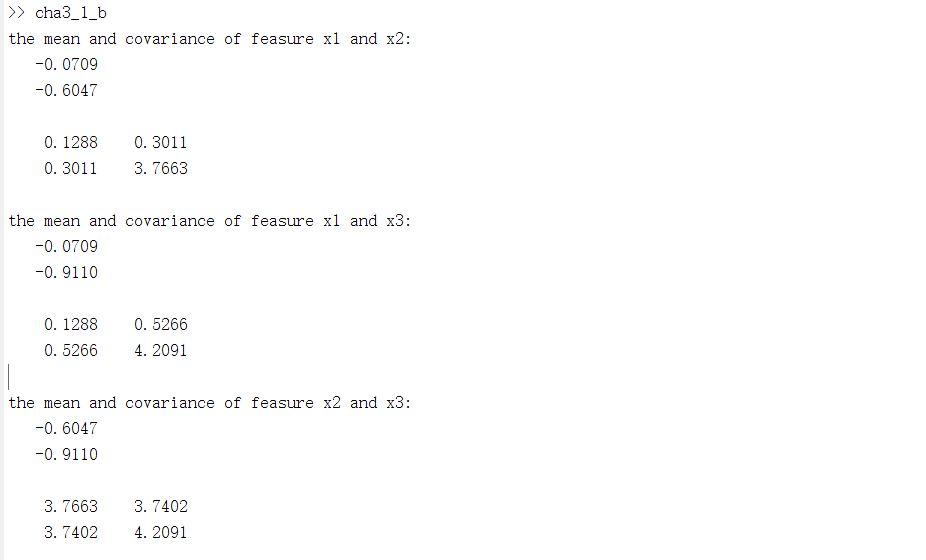通过对比一维二维结果，发现一维均值是二维均值向量里的一个分量，方差却不是。并且二维协方差矩阵里对角的元素代表不同的特征。对于三维的情况（c），同理：
clear;
u=mean(w1',2);
sum=0;
for i=1:size(w1,1);
sum=sum+(w1(i,:)'-u)*(w1(i,:)'-u)';
end
sigma=sum/size(w1,1);
disp('the mean and covariance of w1:');
disp(u);disp(sigma);
运行结果如下：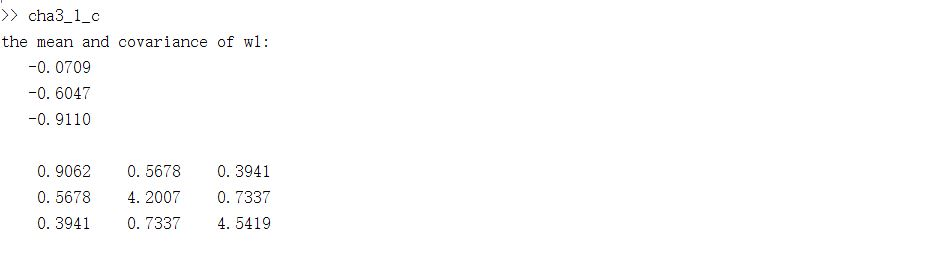对比一维和三维情况，我们发现一维均值正好对应三维均值里每一个分量，一维方差对应三维协方差矩阵中的对角元素。对于（d），由于这个三维模型是可分离的，也就是各个特征之间互相独立不会影响因此它的协方差矩阵是个对角矩阵，对角元素即为各个特征的方差。我们可以直接求取：
load sample_ex3.mat;
x1=w2(:,1);x2=w2(:,2);x3=w2(:,3);
u1=mean(x1);sigma1=mean((x1-u1).^2);
u2=mean(x2);sigma2=mean((x2-u2).^2);
u3=mean(x3);sigma3=mean((x3-u3).^2);
u=[u1;u2;u3];
sigma=[sigma1,0,0;0,sigma2,0;0,0,sigma3];
disp('the mean and covariance of w2:');
disp(u);disp(sigma);
结果如下：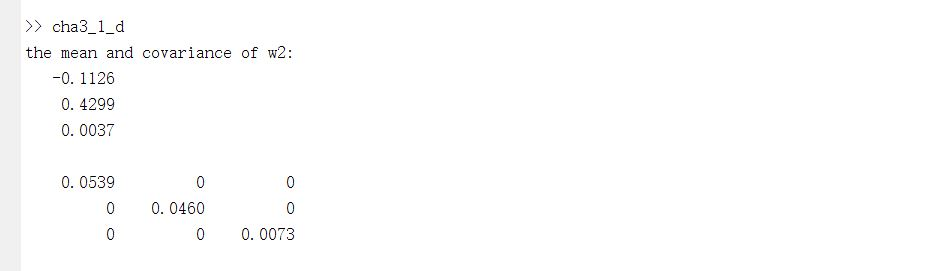分析与体会：
通过对比一维二维结果，发现一维均值是二维均值向量里的一个分量，方差却不是。并且二维协方差矩阵里对角的元素代表不同的特征。对比一维和三维情况，我们发现一维均值正好对应三维均值里每一个分量，一维方差对应三维协方差矩阵中的对角元素。
展开全文机器学习 matlab 数据
• ## 最大似然估计算法

千次阅读 2017-06-22 15:21:46
最大似然估计提供了一种给定观察数据来评估模型参数的方法，即：“模型已定，参数未知”。简单而言，假设我们要统计全国人口的身高，首先假设这个身高服从服从正态分布，但是该分布的均值与方差未知。我们没有人力与...
    最大似然估计提供了一种给定观察数据来评估模型参数的方法，即：“模型已定，参数未知”。简单而言，假设我们要统计全国人口的身高，首先假设这个身高服从服从正态分布，但是该分布的均值与方差未知。我们没有人力与物力去统计全国每个人的身高，但是可以通过采样，获取部分人的身高，然后通过最大似然估计来获取上述假设中的正态分布的均值与方差。
最大似然估计中采样需满足一个很重要的假设，就是所有的采样都是独立同分布的。下面我们具体描述一下最大似然估计：
首先，假设为独立同分布的采样，θ为模型参数,f为我们所使用的模型，遵循我们上述的独立同分布假设。参数为θ的模型f产生上述采样可表示为回到上面的“模型已定，参数未知”的说法，此时，我们已知的为，未知为θ，故似然定义为:在实际应用中常用的是两边取对数，得到公式如下：其中称为对数似然，而称为平均对数似然。而我们平时所称的最大似然为最大的对数平均似然，即：举个别人博客中的例子，假如有一个罐子，里面有黑白两种颜色的球，数目多少不知，两种颜色的比例也不知。我 们想知道罐中白球和黑球的比例，但我们不能把罐中的球全部拿出来数。现在我们可以每次任意从已经摇匀的罐中拿一个球出来，记录球的颜色，然后把拿出来的球 再放回罐中。这个过程可以重复，我们可以用记录的球的颜色来估计罐中黑白球的比例。假如在前面的一百次重复记录中，有七十次是白球，请问罐中白球所占的比例最有可能是多少？很多人马上就有答案了：70%。而其后的理论支撑是什么呢？
我们假设罐中白球的比例是p，那么黑球的比例就是1-p。因为每抽一个球出来，在记录颜色之后，我们把抽出的球放回了罐中并摇匀，所以每次抽出来的球的颜 色服从同一独立分布。这里我们把一次抽出来球的颜色称为一次抽样。题目中在一百次抽样中，七十次是白球的概率是P(Data | M)，这里Data是所有的数据，M是所给出的模型，表示每次抽出来的球是白色的概率为p。如果第一抽样的结果记为x1，第二抽样的结果记为x2... 那么Data = (x1,x2,…,x100)。这样，
P(Data | M)
= P(x1,x2,…,x100|M)
= P(x1|M)P(x2|M)…P(x100|M)
= p^70(1-p)^30.
那么p在取什么值的时候，P(Data |M)的值最大呢？将p^70(1-p)^30对p求导，并其等于零。
70p^69(1-p)^30-p^70*30(1-p)^29=0。
解方程可以得到p=0.7。
在边界点p=0,1，P(Data|M)=0。所以当p=0.7时，P(Data|M)的值最大。这和我们常识中按抽样中的比例来计算的结果是一样的。
假如我们有一组连续变量的采样值（x1,x2,…,xn），我们知道这组数据服从正态分布，标准差已知。请问这个正态分布的期望值为多少时，产生这个已有数据的概率最大？
P(Data | M) = ？
根据公式可得:对μ求导可得,则最大似然估计的结果为μ=(x1+x2+…+xn)/n

由上可知最大似然估计的一般求解过程：
（1） 写出似然函数；
（2） 对似然函数取对数，并整理；
（3） 求导数 ；
（4） 解似然方程

注意：最大似然估计只考虑某个模型能产生某个给定观察序列的概率。而未考虑该模型本身的概率。这点与贝叶斯估计区别。
展开全文优化
• 针对现有各种降噪方法存在的缺点，提出了一种改进标准化最大似然估计的最小描述长度降噪方法。该方法增加编码过程中对集合本身码长计算，降噪中自适应确定降噪阈值。通过仿真信号和实际某轴承故障信号降噪分析，结果...
• 基于分块级的模式噪声,提出一种基于最大似然估计的自适应阈值视频被动取证方法.它采用小波去噪和维纳滤波提取传感器的模式噪声,并通过固定大小的滑动窗口,计算分块级的能量梯度、信噪比和相邻帧相同位置块模式噪声的...
• ## 最大似然估计

千次阅读 2018-10-06 17:42:27
最大似然估计是一种求估计量的方法，它在求估计量的方法中地位是比较高的。那么最大似然估计法就是参数估计中的点估计中的一种方法的，按照变量的类型主要分为离散型随机变量与连续性随机变量。 二、离散型随机变量...
• 最大似然波达方向(DOA)估计具有最优的理论性能,但是存在计算量过大的问题。为了降低最大似然DOA估计的计算量,将参数估计转化为高维非线性函数的优化问题,并提出了一种新的优化算法。首先利用波束形成法对空间谱进行...
•   极大似然估计，我们也把它叫做最大似然估计(Maximum Likelihood Estimation)，英文简称MLE。它是机器学习中常用的一种参数估计方法。它提供了一种给定观测数据来评估模型参数的方法。也就是模型已知，参数未定。...算法 机器学习
• 二话不说咱先抛出一个问题：数据集Data X服从高斯分布，如何推导X的均值和方差 欲解答上述问题，先对问题进行拆解： 1、何为高斯分布，高斯分布概率密度函数是什么 2、用什么方法推导：最大似然估计法，那最大似然...机器学习 高斯分布 算法
• 似然函数似然函数在给定参数的条件下就是观测到一组数据概率密度函数（连续分布）或概率聚集函数（离散分布）在统计学中，似然函数（likelihood function，通常简写为likelihood，似然）是一个非常重要的内容，在非...
• 针对低剂量计算机断层成像(CT)重建的图像产生严重退化的问题,提出一种基于片相似性各项异性扩散和最大似然期望最大化(MLEM)的低剂量CT重建算法。首先,采用基本的MLEM算法对低剂量投影数据进行重建;然后,由于片相似性...
• 我们如何计算高斯分布的参数μ 、σ的最大似然估计？ 我们想要计算的是观测到所有数据的全概率，即所有观测到的数据点的联合概率分布。为此我们需要计算一些条件概率，这可能会很困难。所以这里我们将做出我们的第...
• 最大似然均衡是在使用线性空时编码的 MIMO 系统中估计传输符号的最佳方法（有关理论背景，请参见参考文献 ）。 请注意，对于高阶调制，ML 解码的计算成本可能很高。 zip 文件包含三个 m 文件。 - space_time_...matlab
• 极大似然估计**是神经网络和很多复杂模型得以优化求解的理论基础, 我们今天来学习并试着深入理解极大似然估计的原理和推导, 最后我们对极大似然估计进行3D可视化, 建立一种直观的认识. 要理解极大似然估计是什么, ...
• 用极大似然估计方法估计掷硬币的概率，word版的估计过程，涉及公式，完整版的推导
• ## 最大似然估计方法

千次阅读 2014-04-28 12:36:19
在实际问题中，我们通常得不到问题的概率结构的全部知识，我们只有利用训练样本来 估计 问题所涉及的先验概率和...常用的估计方法最大似然估计、最大后验估计、贝叶斯估计等。 若设x=(x1,…,xn)是来自概率密度
• ## 最大似然参数估计

万次阅读 2017-01-12 17:07:47
最普遍的情况是概率密度函数并不是已知的，在很多的问题中，潜在的概率密度函数必须从可用的数据中估计。例如有时可能知道概率密度函数...这里介绍最大似然参数估计。  考虑一个M类的问题，特征向量服从p(x|wi)，i=1，matlab 模式识别
• 最大似然估计是一种统计方法，它用来求一个样本集的相关概率密度函数的参数。这个方法最早是遗传学家以及统计学家罗纳德·费雪爵士在1912年至1922年间开始使用的。 “似然”是对likelihood 的一种较为贴近文言文的...
• 针对小高比立体匹配当中的亚像素精度和粘合现象问题，提出了一种基于最大似然估计的小基高比立体匹配方法。该方法首先根据混合式窗口选择策略为参考图像中的每一点确定匹配窗口；然后在视差范围内根据规范化互相关...立体匹配 亚像素视差
• 参考： K Vasudevan，“通过频率选择性瑞利衰落信道传输的 Turbo 编码 OFDM 信号的相干检测”，IEEE 信号处理计算和控制国际会议，2013 年 9 月 26 日至 28 日，西姆拉。matlab
• 最大似然估计就是利用已知的样本结果，在使用某个模型的基础上，反推最有可能导致这样的结果的模型参数。 2. 理论阐述 假设我们有一个独立同分布的样本。假设是从某个定义在参数θ上的已知概率密度族p(x|θ)中抽取...统计学 机器学习
• 昨天晚上参加阿里巴巴的实习面试，各种被虐。 伯努利分布的最大似然估计机器学习 伯努利分布...